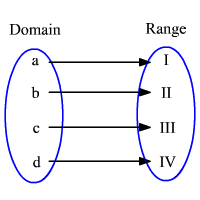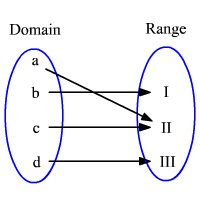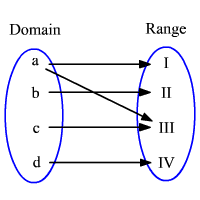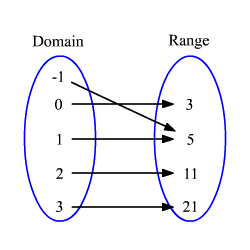# Mapping Diagrams

A function is a special type of relation in which each element of the domain is paired with exactly one element in the range . A mapping shows how the elements are paired. Its like a flow chart for a function, showing the input and output values.A mapping diagram consists of two parallel columns. The first column represents the domain of a function $f$ , and the other column for its range. Lines or arrows are drawn from domain to range, to represent the relation between any two elements.

A function represented by the mapping above in which each element of the range is paired with exactly one element of the domain is called one-to-one mapping.In the mapping, the second element of the range associates with more than one element in the domain. If the element(s) in range that have mapped more than one element in the domain is called many-to-one mapping.In this mapping, the first element in the domain have mapped with more than one element in the range. If one element in the domain mapped with more then one element in the range, the mapping is called one-to-many relation. One-to-many relations are not functions.

Example:

Draw a mapping diagram for the function $f\left(x\right)=2{x}^{2}+3$ in the set of real numbers.

First choose some elements from the domain. Then find the corresponding $y$ -values (range) for the chosen $x$ -values.

The domain of the function is all real numbers. Let $x=-1,0,1,2$ and $3$ .

Substitute these values into the function $f\left(x\right)$ to find its range.

The corresponding $y$ -values (range) are $5,3,5,11$ and $21$ .

Now draw the mapping diagram.